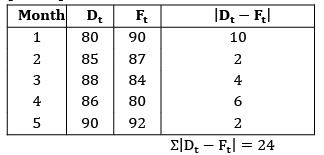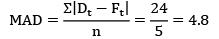Courses

# Test: Forecasting (Level - 1)

## 20 Questions MCQ Test Manufacturing and Industrial Engineering | Test: Forecasting (Level - 1)

Description
This mock test of Test: Forecasting (Level - 1) for Mechanical Engineering helps you for every Mechanical Engineering entrance exam. This contains 20 Multiple Choice Questions for Mechanical Engineering Test: Forecasting (Level - 1) (mcq) to study with solutions a complete question bank. The solved questions answers in this Test: Forecasting (Level - 1) quiz give you a good mix of easy questions and tough questions. Mechanical Engineering students definitely take this Test: Forecasting (Level - 1) exercise for a better result in the exam. You can find other Test: Forecasting (Level - 1) extra questions, long questions & short questions for Mechanical Engineering on EduRev as well by searching above.
QUESTION: 1

### Which one of the following is not a technique of Long Range Forecasting?

Solution: Correlation and Regression method is used for short and medium range forecasting.

QUESTION: 2

Solution:
QUESTION: 3

### Which one of the following is a qualitative technique of demand forecasting?

Solution:
QUESTION: 4

For sales forecasting, pooling of expert opinions is made use of in

Solution:
QUESTION: 5

In an n-month moving average methods for stable demand, the ‘n’ value in a simple moving average method should be

Ans~ B

Solution:
*Answer can only contain numeric values
QUESTION: 6

The actual demand values for 4 months is known. To calculate the forecasted demand value for 5th month using 4 month moving average method, what will be the weightage given to demand values?

Solution: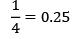QUESTION: 7

In exponential smoothening method the weights are in

Solution:
QUESTION: 8

Which of the following is not a weighted average method?

Solution:
QUESTION: 9

Which one of the following methods can be used for forecasting when a demand pattern is consistently increasing or decreasing?

Ans~ A

Solution:
QUESTION: 10

Which of the following represents forecasting errors?

1. Mean forecast error

2. Mean absolute deviation

3. Tracking signal

Solution:
*Answer can only contain numeric values
QUESTION: 11

Consider the given sales data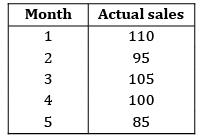The forecast weights are given as 40%, 30%, 20% and 10%. Find the forecasted value for the month of 6 using 4 month weighted moving average method.

Solution: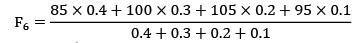= 94.5

QUESTION: 12

Using the exponential smoothing method of forecasting, what will be the forecast for the fourth week if the actual and forecasted demand for the third week is 480 and 500 respectively and α = 0.2?

Solution:

F4 = αd3 + (1 − α)F3

F3 = (0.2)(480) + (0.8)500

= 96 + 400 = 496

QUESTION: 13

The demand for a product in the month of March turned out to be 20 units against an earlier made forecast of 20 units. The actual demand for April and May turned to be 25 and 26 units respectively. What will be the forecast for the month of June, using exponential smoothing method and taking smoothing constant α as 0.2?

Solution:

α = 0.2, DMarch = 20 units DApril = 25 DMay = 26

FMar = 20 units FApril = 20 FMay = 21 FJun =?

FApril = α × DMar + (1 − α) FMar = 0.2 × 20 + 0.8 × 20 = 20

FMay = α × DApril + (1 − α) × FApril = 0.2 × 25 + 0.8 × 20 = 21

FJune = α × DMay + (1 − α) × FMay

FMay = 0.2 × 26 + 0.8 × 21 = 22 units

QUESTION: 14

Which of the following curve represents variation of weights with respect to time for exponential smoothening method?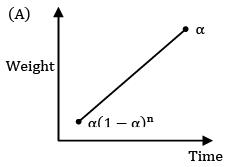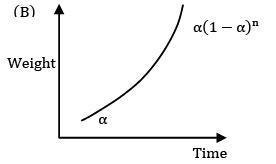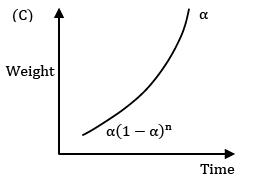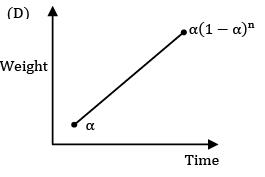Solution:
QUESTION: 15

For stable demand the value of α should be

Solution: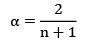For stable demand ‘n’ value should be more

⇒ α will be less.

QUESTION: 16

The sale of cars in a shop for four years is given as 102, 98, 108, 115. The sales follow exponentially smoothening average method with a smoothing factor of 0.2. The number of sales in the subsequent month will be (approximately):

Solution:

Ft+1 = αDt + α(1 − α)Dt−1 + α(1 − α)2Dt−2+ α(1 − α)3Dt−3

= 0.2(115) + 0.2(1 − 0.2) × 108

+ 0.2(1 − 0.2)2 × 98

+ 0.2(1 − 0.2)3 × 102

= 63.26

*Answer can only contain numeric values
QUESTION: 17

The actual demand for a product from January to June is known. The actual demand and forecasted demand for the month of June is 90 and 80. Find the forecasted demand for the month of July.

Solution:

Ft+1 = Ft + α(Dt − Ft )

FJuly = FJune + α(DJune − FJune )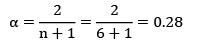FJuly = 80 + 0.28(90 − 80)

= 82.8

QUESTION: 18

Which of the following forecast error is not used widely because of null effect?

Solution:
QUESTION: 19

Which of the following method can be used for the following demand data?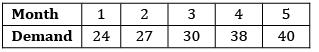Solution: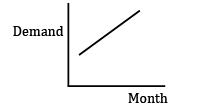The demand is having an increasing pattern. So use regression analysis

QUESTION: 20

Consider the following demand data values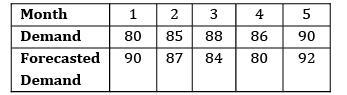The mean absolute deviation is

Solution: